# Test Prep Plan - Take a practice test

Take this practice test to check your existing knowledge of the course material. We'll review your answers and create a Test Prep Plan for you based on your results.
How Test Prep Plans work
1
2Based on your results, we'll create a customized Test Prep Plan just for you!
3Study smarter
Study more effectively: skip concepts you already know and focus on what you still need to learn.

Exam Instructions:

Choose your answers to the questions and click 'Next' to see the next set of questions. You can skip questions if you would like and come back to them later with the yellow "Go To First Skipped Question" button. When you have completed the practice exam, a green submit button will appear. Click it to see your results. Good luck!

### Page 1

#### Question 3 3. What is the vertex form of the following equation?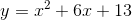### Page 2

#### Question 6 6. What inequality symbol is represented by the red dashed line?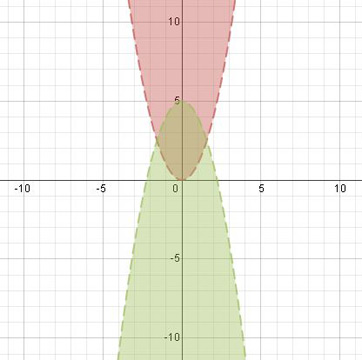#### Question 8 8. What would the first step be in completing the square of the following equation?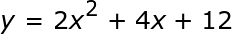### Page 3

#### Question 11 11. If the equation below told you a ball was 'h' feet in the air after 't' seconds, what would be the first step to finding out when it hit the ground?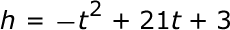#### Question 13 13. Describe the roots of the following quadratic expression?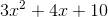#### Question 15 15. What is the intercept form of the equation for the parabola: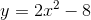### Page 4

#### Question 16 16. Find the value for c that must be added to both sides, and rewrite the equation in the vertex form.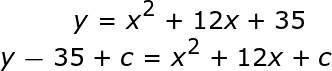#### Question 17 17. What is the equation of the following graph?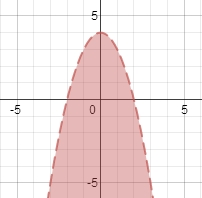#### Question 19 19. What is the vertex form of the following equation?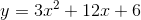#### Question 20 20. What is the b-value of the quadratic equation below?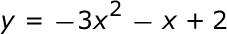### Page 5

#### Question 21 21. Where does the axis of symmetry cross the x-axis for a parabola described by the equation y = -2(x-4)(x+2)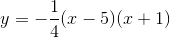#### Question 25 25. What is the intercept form of the standard equation: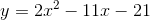### Page 6

#### Question 26 26. Factor the following polynomial: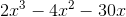#### Question 27 27. Solve the following polynomial using the quadratic equation: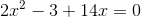#### Question 30 30. Factor the following polynomial: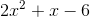#### Quadratic Functions Chapter Exam Instructions

Choose your answers to the questions and click 'Next' to see the next set of questions. You can skip questions if you would like and come back to them later with the yellow "Go To First Skipped Question" button. When you have completed the practice exam, a green submit button will appear. Click it to see your results. Good luck!

Support